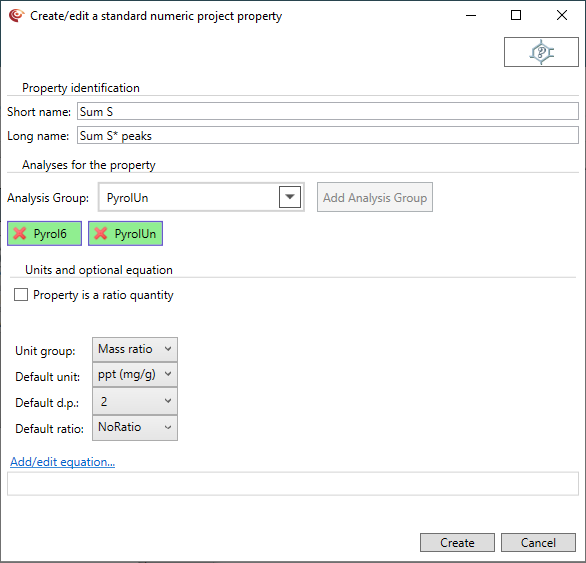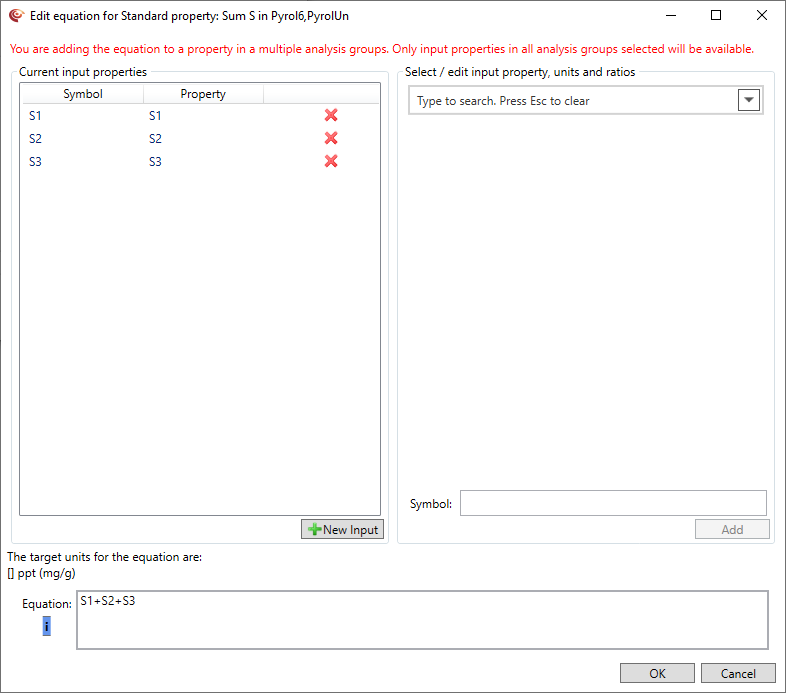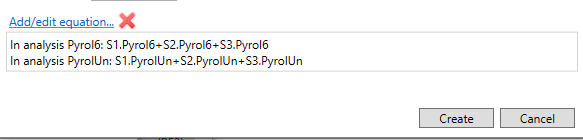# Creating Project Property equations

#### Overview

When defining equations it can be helpful to think of two types. Simple equations that involve some arithmetic on several properties, for example a sum of several properties, and ratio equations, that typically define the ratio of two or more properties. You will need to define the details, including units and where relevant ratio types for all input properties, and you also need to define the target units - all ratio equations are assumed to be written in a/b form. You can share your new project property equations with colleagues whenever you export them with any templates. Project properties are designed to enable you to create your own properties and can be assigned new equations to help you calculate new ratios or other quantities. Equations can be as complex as you wish, and can take in other project properties as inputs, and will auto-evaluate.

Usage:  Data --> New project property...    Add equation for property...

#### How to use in practice

##### Creating simple equationsFirst you need to define the new property that will be the target of the equation. You will need to consider appropriate units for this target. p:IGI+ has a complete unit system, but will not check that the units are sensible - you need to do this.  In general if adding / subtracting properties the units for the result should be the same as the units for the inputs, and if dividing things with the same units the result will be unitless, although for some equations a mass, volume or mol fraction might be appropriate.

This will bring up the equation definition dialogue. This allows you to select a number of properties and combine these in an expression.In the right hand panel you can search for and select properties, as usual in the system. You can change the unit (and where relevant the ratio) to be used for these input properties. p:IGI+ will take care of all unit conversions as the equation is evaluated. You can also define the symbol you want to use for this property when typing the equation. By default you get the property short name, but you can replace this with any name you like.

Clicking the Add button adds this input to the list of input properties on the left and to the equation with an addition. You can remove any properties you don't need using the red cross, or can edit the symbols of properties by clicking on the row and making the change in the right hand panel and selecting the Update button. If you are editing and decide you need to add another new property, notice that the New Input button is now active and can be clicked to return to a blank property selector with which you can add another new variable.

Once you are happy with the inputs you have selected you can start writing the equation. You type the equation as plain text - the equation system will try and parse the text as soon as you pause typing and will tell you whether the equation is valid. There is a blue "i" button for further information on how to write equations - clicking this brings up a window with advice on how to write equations.

Writing equations is designed to work a bit like the way you define equations in Excel, but simpler. Having given the properties symbols you can now use those symbols to write the equation. Let's imagine we have called our properties X, Y and Z for now. Equations can involve:

• Operations: + - * / ^
• Brackets: ()
• Functions: log() ln() exp() sqrt() abs()
• Special functions: exists{} use{ A else B}

Equations take the form of expressions which combine the above and symbols for example:

• X+Y/100 - this will add X to (Y divided by 100).
• (X+Y)/(Z-2) - this will add X to Y, then divide this by (Z minus 2). Note that brackets can be nested to ensure the right order of evaluation.
• exp(-Y/100)*ln(Z) - this will calculate the exponent of -Y/100 and then multiply this by the natural logarithm of Z.
• X+Y+exists{Z} - this will add X and Y, and if present Z. If Z is missing the exists keyword will replace it by a zero.
• use{X else Y}+Z - this will add X to Z if X has a value, if not it will try and add Y to Z.

The exists{} special function allows you do include inputs which might often not be measured, but when they are should be included, but means that if they are missing the equation is still evaluated. When the term inside the exists{} special function is involved in addition or subtraction, if the value is missing it is assumed to be zero, when used in multiplication or division it is assumed to be one.

The use{X else Y} can be used if you prefer to use a particular property when it is available, but if not then another can be substituted. In addition you can use the pre-defined Any properties, which have their own substitution rules which will be used in the equations.

Once you are happy with the equation, and are also happy you have set the target unit for the equation you can press the OK button to add the equation to your new property. Note that for any ratio quantities you should always write the equation as a/b, even if by default you want to see the result as a/(a+b), since you can set the default ratio separately. Writing equations as a/b keeps them compact. When you have pressed OK you will be returned to the project property dialogue, but will notice that it now has an equation being displayed as shown below.You can still edit the equation by selecting the Add equation for property... link or remove it using the red x. Once you are happy with the project property being created, including the equation, you press the Create button. You might notice a pause in the application at this point, while p:IGI+ updates the database, adds the equation to the equation system (which is a complicated job!) and then evaluates the equation on all possible samples. In large data sets this pause might be quite noticeable.

Note that the creation of project properties and equations can be undone. You can also delete the project properties later if necessary.